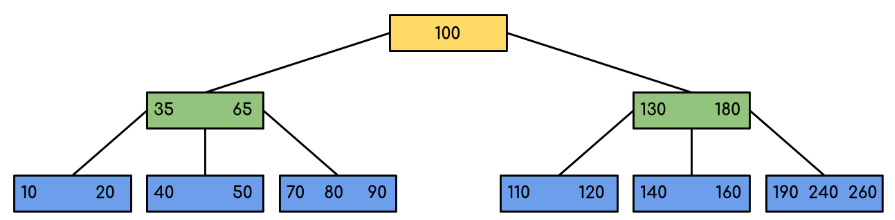B 树是为磁盘或其他直接存取的辅助存储设备而设计的一种平衡搜索树。B 树类似于红黑树，但它们在降低磁盘 I/O 操作数方面要更好一点，比如很多数据库都使用 B 树或 B 树的变种来存储信息。## B 树 #

B 树有的地方也称 B-tree，但不要叫“B 减树”。B 树与红黑树的不同之处在于 B 树的结点可以有从数个到数千个很多孩子，也就是说，一个 B 树的“分支因子”可以相当大，它通常依赖于所使用的磁盘单元的特性。

### 性质 #

1. 每个结点 x 有以下属性：
1. x.n，当前存储在结点 x 中的关键字（非子结点概念）个数。
2. x.n 个关键字本身 $x.key_1$、$x.key_2$、$x.key_3$ … $x.key_n$ 以非降序存放，使得 $x.key_1$ <= $x.key_2$ <= … <= $x.key_n$
3. x.leaf ，一个布尔值，如果 x 是叶结点，则为 True；如果 x 为内部结点，则为 False
2. 每个内部结点 x 还包含 x.n+1 个指向其孩子的指针 x.c1x.c2x.c3、$x.c_{x.n+1}$ ，叶子结点没有孩子，所以它们的 $c_i$ 属性没有定义。
3. 关键字 $x.key_i$ 对存储在各子树中的关键字范围加以分割：如果 $k_i$ 为任意一个存储在以 $x.c_i$ 为根的子树中的关键字，那么 $k_1$ <= $x.key_1$ <= $k_2$ <= $x.key_2$ <= … <= $x.key_{x.n}$ <= $k_{x.n+1}$ 。
4. 每个叶结点具有相同的深度，即树的高度 h
5. 每个结点所包含的关键字个数有上界和下界，用一个被称为 B 树的最小度数的固定整数 t>=2 来表示这些界。
1. 除了根结点以外的每个结点必须至少有 t-1 个关键字，因此，除了根结点以外的每个内部结点至少有 t 个孩子，如果树非空，根结点至少有一个关键字。
2. 每个结点至多可包含 2t-1 个关键字，因此，一个内部结点至多可有 2t 个孩子，当一个结点恰好有 2t-1 个关键字时，称该结点是满的（full）。

$$h <= log_t{\frac{n+1}{2}}$$

## B+ 树 #

B+ 树是 B 树的一个变种，它把所有的卫星数据都存储在叶结点中，内部结点只存放关键字和孩子指针，因此最大化了内部结点的分支因子。